In :
%matplotlib inline
import matplotlib
import matplotlib.pyplot as plt
import pandas as pd

plt.style.use('ggplot')


## Getting the data¶

From Yahoo Finance's Historical Prices: Apple Inc. (AAPL) stock: http://finance.yahoo.com/q/hp?s=AAPL

Direct link to CSV for 2012-01-01 to 2015-05-01:

http://real-chart.finance.yahoo.com/table.csv?s=AAPL&a=00&b=1&c=2013&d=04&e=1&f=2015&g=d

The parse_dates argument takes in a list of column numbers, in which we can use to tell pandas which columns are meant to be dates. In this case, it's the first column, i.e. the 0th:

In :
csvurl = "http://real-chart.finance.yahoo.com/table.csv?s=AAPL&a=00&b=1&c=2010&d=04&e=1&f=2015&g=d"
prices = pd.read_csv(csvurl, parse_dates = )

In :
prices.head()

Out:
Date Open High Low Close Volume Adj Close
0 2015-05-01 126.10000 130.13000 125.30000 128.95000 57195000 128.95000
1 2015-04-30 128.64000 128.64000 124.58000 125.15000 82475900 125.15000
2 2015-04-29 130.16000 131.59000 128.30000 128.64000 62410800 128.64000
3 2015-04-28 134.46001 134.53999 129.57001 130.56000 118580700 130.56000
4 2015-04-27 132.31000 133.13000 131.14999 132.64999 84783100 132.64999

## Making a chart¶

In :
fig, ax = plt.subplots()


Out:
[<matplotlib.lines.Line2D at 0x10d441f98>]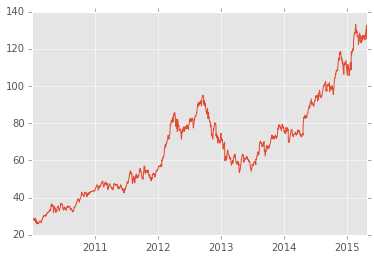## Reshaping the data¶

Use the DataFrame method set_index() to create a DataFrame that uses the "Date" column as an index:

### Tidy the chart¶

Use the pandas Timeseries to generate ticks for the x-axis. Here's a list of aliases for time periods -- 'BQ' stands for "business quarter end", for example. I've decided to use '6m' for "6 months".

In :
# fig, ax = plt.subplots()
# redo the axes
# fix the ticks
# ax.set_xticks(pd.date_range('2010-01-01', '2015-05-01', freq = '6m'))
# ax.xaxis.set_major_formatter(matplotlib.dates.DateFormatter('%b %Y'))

In :
dprices = prices.set_index('Date')

Out:
Open High Low Close Volume Adj Close
Date
2015-05-01 126.10000 130.13000 125.30000 128.95000 57195000 128.95000
2015-04-30 128.64000 128.64000 124.58000 125.15000 82475900 125.15000
2015-04-29 130.16000 131.59000 128.30000 128.64000 62410800 128.64000
2015-04-28 134.46001 134.53999 129.57001 130.56000 118580700 130.56000
2015-04-27 132.31000 133.13000 131.14999 132.64999 84783100 132.64999

This doesn't change anything about the number of rows or what they contain (other than that the "Date" column has been moved out of the columns and into the index).

With "Date" no longer an actual column, the plot() call will refer to the index attribute of the DataFrame. But the visual output should be exactly the same as before.

In :
fig, ax = plt.subplots()

Out:
[<matplotlib.lines.Line2D at 0x10ce95278>]### Resampling¶

In the timespan of 2010 to 2015, there are more than 1,300 datapoints. When looking at stock trends over a half-decade period, is it necessary to see each day represented on the graph? If we reduce the granularity of the data, we can still see the same trends in a clearer, less jagged graph, and the loss in precision is irrelevant.

Let's see what the data looks like when charted as weekly periods.

In terms of programmatic logic, this is a kind of groupby call, in which we group the index (i.e. the "Date") by its year-month representation, e.g. "2014-02".

However, switching up the frequency in a time-series is such a common scenario that pandas has a helpful convenience function named resample().

The only required argument for resample() is a string that specifies the frequency of sampling, such as 'W' for weekly and 'A' for annual (a list of aliases can be found here). We can specify an optional how argument, for example, a string such as 'max' or 'first', to get the max value or the first value, respectively, of every week. The default value of how is 'mean'.

To resample the data such that we get the weekly average of the stock price data:

In :
wkprices = dprices.resample('W', how = 'mean')  # 'mean' is default, but we'll be explicit here

Out:
Open High Low Close Volume Adj Close
Date
2010-01-10 212.891994 213.863990 210.898000 212.384008 128626820 28.618940
2010-01-17 210.179996 211.151992 206.771994 208.767998 134477140 28.131680
2010-01-24 210.525002 212.887525 205.277482 208.147502 177005150 28.048070
2010-01-31 204.263988 207.338002 198.249994 201.647990 353741640 27.172254
2010-02-07 194.562006 197.377992 192.304002 195.465996 183575280 26.339226

Let's chart the wkprices data to see what it looks like; the result is slightly less jagged with no real loss in being able to interpret the trends:

In :
fig, ax = plt.subplots()

Out:
[<matplotlib.lines.Line2D at 0x10d33df28>]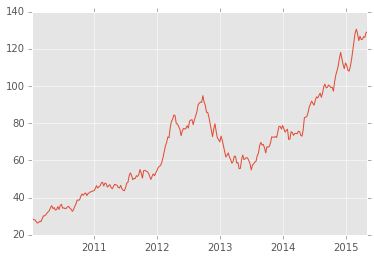Let's break the data down by monthly averages. Then let's display the three data series on the same chart to see how the trendlines compare:

In :
mthprices = dprices.resample('M', how = 'mean')

fig, ax = plt.subplots()
ax.plot(mthprices.index, mthprices['Adj Close'], linewidth = 4, color = 'yellow', )
ax.plot(wkprices.index, wkprices['Adj Close'], linewidth = 4, color = 'blue', alpha = 0.4, linestyle = '--')
ax.plot(dprices.index, dprices['Adj Close'], linewidth = 1, color = 'red', linestyle = ':')

Out:
[<matplotlib.lines.Line2D at 0x10ea22ef0>]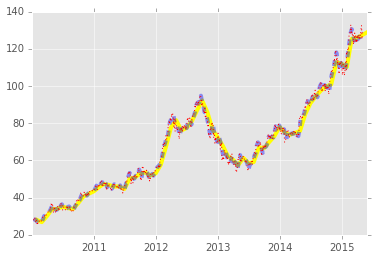It's an ugly graph, but the point is that when looking at 5+ year timespan, daily values end up being noise. The monthly averages seem to work just fine for our purposes so we'll stick to that.

## Making a nice chart¶

• Set the y-axis to a start at 0
• Clean up tick marks
In :
fig, ax = plt.subplots()
ax.set_ylim(ymin = 0)
ax.set_ylabel('Adjusted closing price, monthly average', fontsize=10)
ax.set_title('Apple (AAPL) stock')
# remove the ticks that are along the top and right side of the chart
ax.xaxis.set_ticks_position('bottom')
ax.yaxis.set_ticks_position('left')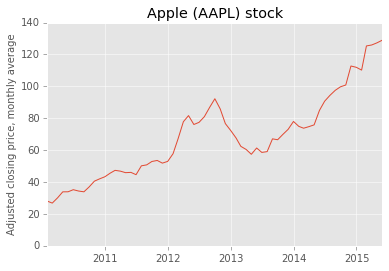## Adding context with another data series¶

Let's add computing rivals Dell (DELL), Hewlett-Packard (HPQ), Microsoft (MSFT), and Sony (SNE) to the analysis. And let's expand it to 1990.

In :
from collections import OrderedDict
NAMES = ['AAPL', 'DELL', 'HPQ', 'MSFT', 'SNE']
COLORS = ['red', 'gray', 'orange', 'green', 'blue']
data = OrderedDict({})


#### Getting the data¶

We'll have to redo the initial data collection from the Yahoo Finance historical price data: http://finance.yahoo.com/q/hp?s=AAPL.

This is the same pattern as before, except in a for-loop. I've decided to create a dictionary called data that will hold a DataFrame for each company label. I could've arranged it to have one large DataFrame and then added a new column for the company label. But this works just fine.

In :
for n in NAMES:
csvurl = "http://real-chart.finance.yahoo.com/table.csv?s=%s&a=00&b=1&c=1990&d=04&e=1&f=2015&g=d" % n
prices = pd.read_csv(csvurl, parse_dates = , index_col = 0)
data[n] = prices.resample('M')


### Each series gets its own chart¶

In :
for name, prices in data.items():
fig, ax = plt.subplots()
ax.set_title(name)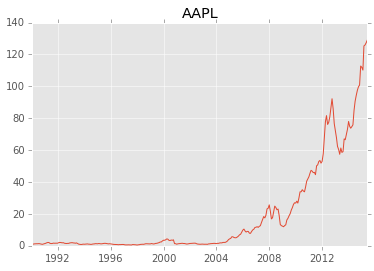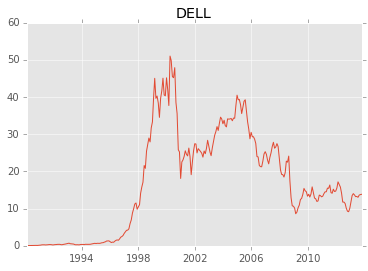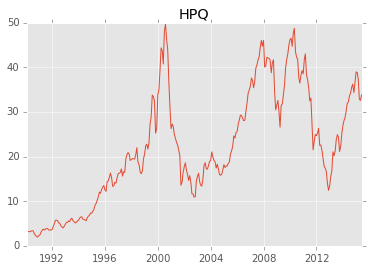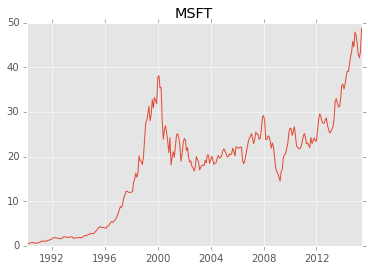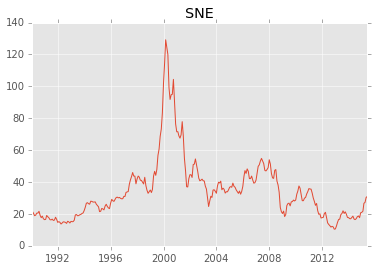This is the laziest, most un-useful way to compare the data: make one chart for each company. One of the main problems with making separate charts is that pandas will autoscale the axes for each series; the y-scale is different for each company, which is severely misleading as the top value for HPQ is not at all the same as it is for AAPL, but you wouldn't know it without looking at the fine print of the labels.

### Multiple series on one chart¶

In :
fig, ax = plt.subplots()
for i, (name, prices) in enumerate(data.items()):
ax.plot(prices.index, prices['Adj Close'], label = name, color = COLORS[i])

ax.legend(loc = 'upper left')

Out:
<matplotlib.legend.Legend at 0x1111ced68>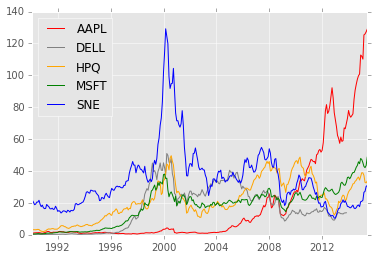By specifying the label arugment in each plot() call, the subsequent legend() call can properly match up each series to its color. The matplotlib docs have much more detail on how to position and format legends.

### Using subplots¶

So far, we've been using the subplots() call even though we've only constructed and rendered one chart at a time. By passing in multiple arguments, we can specify a grid of axes to plot on.

(Check out this tutorial example from the docs of how to use subplots())

In :
fig, axes = plt.subplots(5)
for i, (name, prices) in enumerate(data.items()):
ax = axes[i]
ax.set_title(name)
ax.plot(prices.index, prices['Adj Close'], label = name, color = COLORS[i])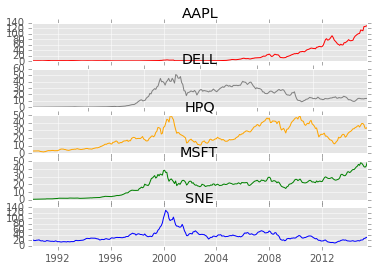In :
fig, axes = plt.subplots(5, sharex=True)
for i, (name, prices) in enumerate(data.items()):
ax = axes[i]
ax.set_title(name)
ax.plot(prices.index, prices['Adj Close'], color = COLORS[i])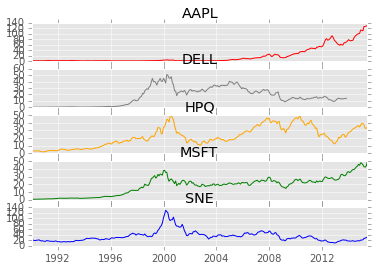In :
fig, axes = plt.subplots(5, sharex=True, sharey=True)
for i, (name, prices) in enumerate(data.items()):
ax = axes[i]
ax.set_title(name)
ax.plot(prices.index, prices['Adj Close'], color = COLORS[i])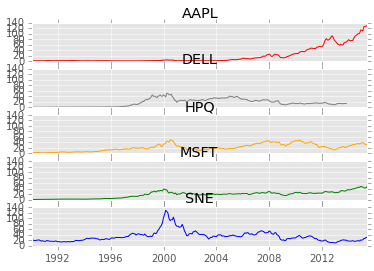In :
fig, axes = plt.subplots(1, 5, sharex=True, sharey=True)
for i, (name, prices) in enumerate(data.items()):
ax = axes[i]
ax.set_title(name)
ax.plot(prices.index, prices['Adj Close'],  color = COLORS[i])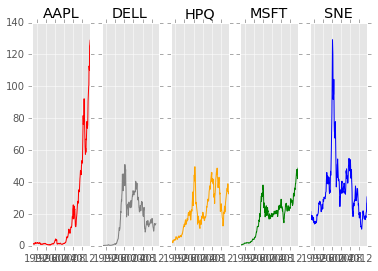In :
fig, axes = plt.subplots(2, 3, sharex=True, sharey=True)
for i, (name, prices) in enumerate(data.items()):
row_num = i / 2
col_num = i % 2
ax = axes[col_num][row_num]
ax.set_title(name)
ax.plot(prices.index, prices['Adj Close'],  color = COLORS[i])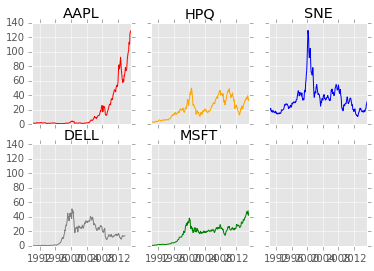In :
fig, axes = plt.subplots(2, 3, sharex=True, sharey=True)
for i, (name, prices) in enumerate(data.items()):
row_num = i / 2
col_num = i % 2
ax = axes[col_num][row_num]
ax.set_title(name)
ppchange = prices.pct_change()
ax.scatter(ppchange.index, ppchange['Adj Close'] * 100,  color = COLORS[i], s=1)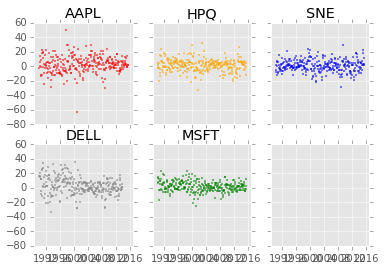In :
fig, ax = plt.subplots()
for i, (name, prices) in enumerate(data.items()):
xprices = prices.resample('A')
ppchange = xprices.pct_change()
ax.scatter(ppchange.index.year, ppchange['Adj Close'] * 100,  color = COLORS[i], s=10, label = name)
plt.legend(loc = 'upper left')

Out:
<matplotlib.legend.Legend at 0x10fe96048>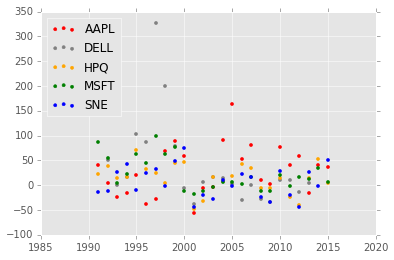In [ ]: